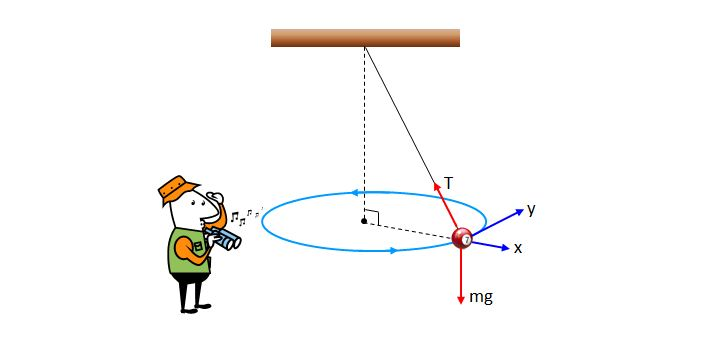# circular pendulumThe above shows a pendulum which is in uniform circular motion on a horizontal plane, hanging on a string from the ceiling. In the picture, $mg$ denotes the gravitational force, and $T$ denotes the tensile force of the string. Which of the following statements is correct in this connection? (Ignore air resistance and the mass of the string.)

a) The magnitude of the tensile force $T$ is greater than that of the gravitational force $mg$.

b) If we cut off the string right at this moment, then the pendulum will move as if it is thrown to the $x$ direction.

c) The direction of the net force acting on the pendulum is the $y$ direction.

×